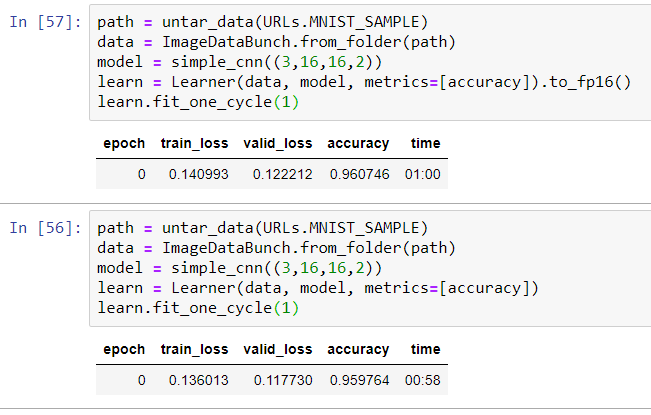# Verify model and dataset float16 type - Planet

Hi,
I am working with the Planet dataset. It does not fit my GPU’s RAM (6gb - 1660ti), so I am trying to use the float16 format by using the following code:

learn = cnn_learner(data, arch, metrics=[acc_02, f_score]).to_fp16()

data = (src.transform(tfms, size=256)
.databunch(bs = 20).normalize(imagenet_stats))